# 12.2 The hyperbola  (Page 9/13)

 Page 9 / 13

$\frac{{\left(y-6\right)}^{2}}{36}-\frac{{\left(x+1\right)}^{2}}{16}=1$

$\frac{{\left(x-2\right)}^{2}}{49}-\frac{{\left(y+7\right)}^{2}}{49}=1$

$\frac{{\left(x-2\right)}^{2}}{{7}^{2}}-\frac{{\left(y+7\right)}^{2}}{{7}^{2}}=1;\text{\hspace{0.17em}}$ vertices: $\text{\hspace{0.17em}}\left(9,-7\right),\left(-5,-7\right);\text{\hspace{0.17em}}$ foci: $\text{\hspace{0.17em}}\left(2+7\sqrt{2},-7\right),\left(2-7\sqrt{2},-7\right);\text{\hspace{0.17em}}$ asymptotes: $\text{\hspace{0.17em}}y=x-9,y=-x-5$

$4{x}^{2}-8x-9{y}^{2}-72y+112=0$

$-9{x}^{2}-54x+9{y}^{2}-54y+81=0$

$\frac{{\left(x+3\right)}^{2}}{{3}^{2}}-\frac{{\left(y-3\right)}^{2}}{{3}^{2}}=1;\text{\hspace{0.17em}}$ vertices: $\text{\hspace{0.17em}}\left(0,3\right),\left(-6,3\right);\text{\hspace{0.17em}}$ foci: $\text{\hspace{0.17em}}\left(-3+3\sqrt{2},1\right),\left(-3-3\sqrt{2},1\right);\text{\hspace{0.17em}}$ asymptotes: $\text{\hspace{0.17em}}y=x+6,y=-x$

$4{x}^{2}-24x-36{y}^{2}-360y+864=0$

$-4{x}^{2}+24x+16{y}^{2}-128y+156=0$

$\frac{{\left(y-4\right)}^{2}}{{2}^{2}}-\frac{{\left(x-3\right)}^{2}}{{4}^{2}}=1;\text{\hspace{0.17em}}$ vertices: $\text{\hspace{0.17em}}\left(3,6\right),\left(3,2\right);\text{\hspace{0.17em}}$ foci: $\text{\hspace{0.17em}}\left(3,4+2\sqrt{5}\right),\left(3,4-2\sqrt{5}\right);\text{\hspace{0.17em}}$ asymptotes: $\text{\hspace{0.17em}}y=\frac{1}{2}\left(x-3\right)+4,y=-\frac{1}{2}\left(x-3\right)+4$

$-4{x}^{2}+40x+25{y}^{2}-100y+100=0$

${x}^{2}+2x-100{y}^{2}-1000y+2401=0$

$\frac{{\left(y+5\right)}^{2}}{{7}^{2}}-\frac{{\left(x+1\right)}^{2}}{{70}^{2}}=1;\text{\hspace{0.17em}}$ vertices: $\text{\hspace{0.17em}}\left(-1,2\right),\left(-1,-12\right);\text{\hspace{0.17em}}$ foci: $\text{\hspace{0.17em}}\left(-1,-5+7\sqrt{101}\right),\left(-1,-5-7\sqrt{101}\right);\text{\hspace{0.17em}}$ asymptotes: $\text{\hspace{0.17em}}y=\frac{1}{10}\left(x+1\right)-5,y=-\frac{1}{10}\left(x+1\right)-5$

$-9{x}^{2}+72x+16{y}^{2}+16y+4=0$

$4{x}^{2}+24x-25{y}^{2}+200y-464=0$

$\frac{{\left(x+3\right)}^{2}}{{5}^{2}}-\frac{{\left(y-4\right)}^{2}}{{2}^{2}}=1;\text{\hspace{0.17em}}$ vertices: $\text{\hspace{0.17em}}\left(2,4\right),\left(-8,4\right);\text{\hspace{0.17em}}$ foci: $\text{\hspace{0.17em}}\left(-3+\sqrt{29},4\right),\left(-3-\sqrt{29},4\right);\text{\hspace{0.17em}}$ asymptotes: $\text{\hspace{0.17em}}y=\frac{2}{5}\left(x+3\right)+4,y=-\frac{2}{5}\left(x+3\right)+4$

For the following exercises, find the equations of the asymptotes for each hyperbola.

$\frac{{y}^{2}}{{3}^{2}}-\frac{{x}^{2}}{{3}^{2}}=1$

$\frac{{\left(x-3\right)}^{2}}{{5}^{2}}-\frac{{\left(y+4\right)}^{2}}{{2}^{2}}=1$

$y=\frac{2}{5}\left(x-3\right)-4,y=-\frac{2}{5}\left(x-3\right)-4$

$\frac{{\left(y-3\right)}^{2}}{{3}^{2}}-\frac{{\left(x+5\right)}^{2}}{{6}^{2}}=1$

$9{x}^{2}-18x-16{y}^{2}+32y-151=0$

$y=\frac{3}{4}\left(x-1\right)+1,y=-\frac{3}{4}\left(x-1\right)+1$

$16{y}^{2}+96y-4{x}^{2}+16x+112=0$

## Graphical

For the following exercises, sketch a graph of the hyperbola, labeling vertices and foci.

$\frac{{x}^{2}}{49}-\frac{{y}^{2}}{16}=1$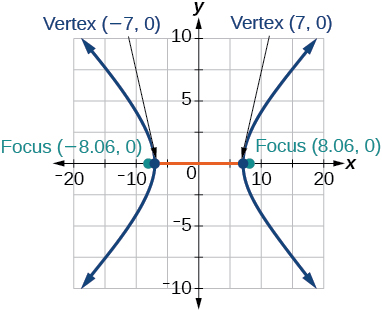$\frac{{x}^{2}}{64}-\frac{{y}^{2}}{4}=1$

$\frac{{y}^{2}}{9}-\frac{{x}^{2}}{25}=1$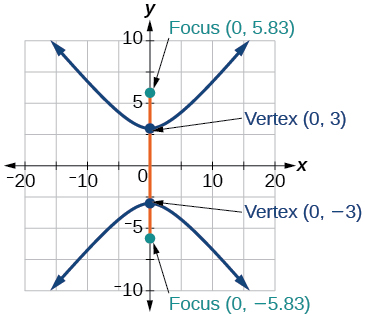$81{x}^{2}-9{y}^{2}=1$

$\frac{{\left(y+5\right)}^{2}}{9}-\frac{{\left(x-4\right)}^{2}}{25}=1$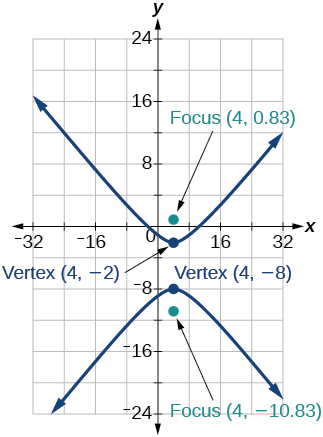$\frac{{\left(x-2\right)}^{2}}{8}-\frac{{\left(y+3\right)}^{2}}{27}=1$

$\frac{{\left(y-3\right)}^{2}}{9}-\frac{{\left(x-3\right)}^{2}}{9}=1$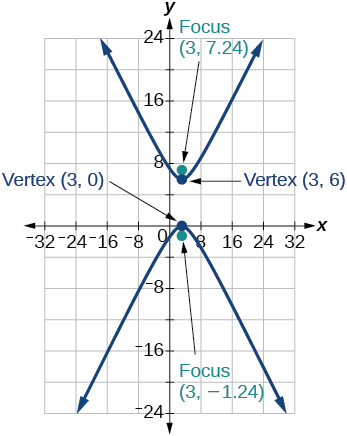$-4{x}^{2}-8x+16{y}^{2}-32y-52=0$

${x}^{2}-8x-25{y}^{2}-100y-109=0$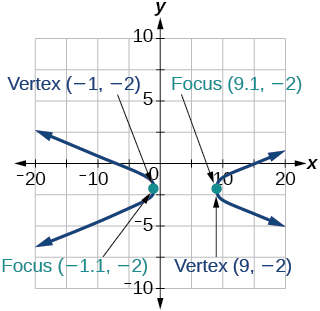$-{x}^{2}+8x+4{y}^{2}-40y+88=0$

$64{x}^{2}+128x-9{y}^{2}-72y-656=0$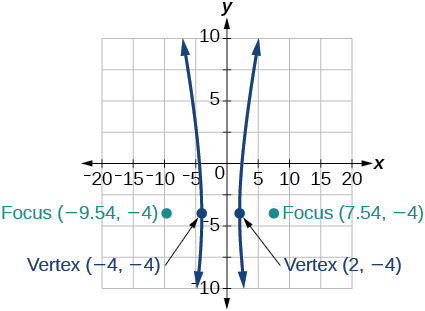$16{x}^{2}+64x-4{y}^{2}-8y-4=0$

$-100{x}^{2}+1000x+{y}^{2}-10y-2575=0$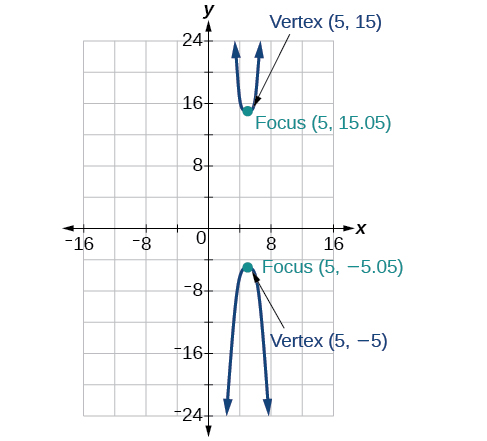$4{x}^{2}+16x-4{y}^{2}+16y+16=0$

For the following exercises, given information about the graph of the hyperbola, find its equation.

Vertices at $\text{\hspace{0.17em}}\left(3,0\right)\text{\hspace{0.17em}}$ and $\text{\hspace{0.17em}}\left(-3,0\right)\text{\hspace{0.17em}}$ and one focus at $\text{\hspace{0.17em}}\left(5,0\right).$

$\frac{{x}^{2}}{9}-\frac{{y}^{2}}{16}=1$

Vertices at $\text{\hspace{0.17em}}\left(0,6\right)\text{\hspace{0.17em}}$ and $\text{\hspace{0.17em}}\left(0,-6\right)\text{\hspace{0.17em}}$ and one focus at $\text{\hspace{0.17em}}\left(0,-8\right).$

Vertices at $\text{\hspace{0.17em}}\left(1,1\right)\text{\hspace{0.17em}}$ and $\text{\hspace{0.17em}}\left(11,1\right)\text{\hspace{0.17em}}$ and one focus at $\text{\hspace{0.17em}}\left(12,1\right).$

$\frac{{\left(x-6\right)}^{2}}{25}-\frac{{\left(y-1\right)}^{2}}{11}=1$

Center: $\text{\hspace{0.17em}}\left(0,0\right);$ vertex: $\text{\hspace{0.17em}}\left(0,-13\right);$ one focus: $\text{\hspace{0.17em}}\left(0,\sqrt{313}\right).$

Center: $\text{\hspace{0.17em}}\left(4,2\right);$ vertex: $\text{\hspace{0.17em}}\left(9,2\right);$ one focus: $\text{\hspace{0.17em}}\left(4+\sqrt{26},2\right).$

$\frac{{\left(x-4\right)}^{2}}{25}-\frac{{\left(y-2\right)}^{2}}{1}=1$

Center: $\text{\hspace{0.17em}}\left(3,5\right);\text{\hspace{0.17em}}$ vertex: $\text{\hspace{0.17em}}\left(3,11\right);\text{\hspace{0.17em}}$ one focus: $\text{\hspace{0.17em}}\left(3,5+2\sqrt{10}\right).$

For the following exercises, given the graph of the hyperbola, find its equation.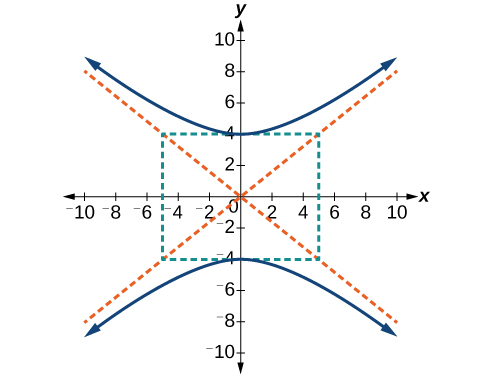$\frac{{y}^{2}}{16}-\frac{{x}^{2}}{25}=1$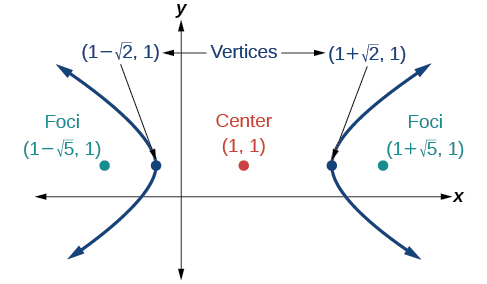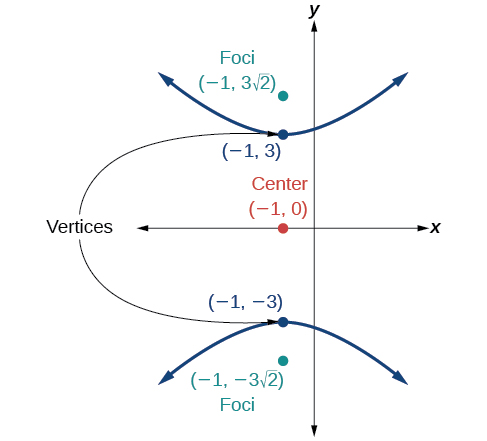$\frac{{y}^{2}}{9}-\frac{{\left(x+1\right)}^{2}}{9}=1$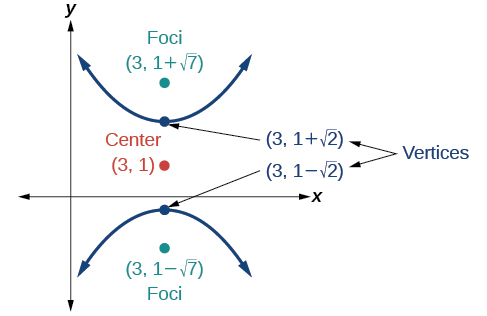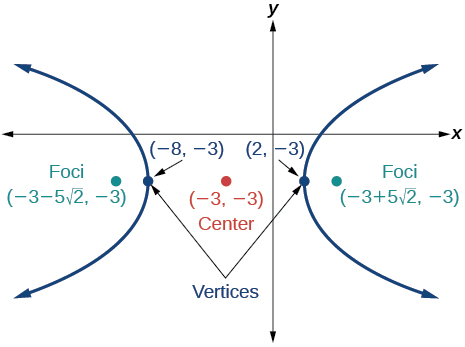$\frac{{\left(x+3\right)}^{2}}{25}-\frac{{\left(y+3\right)}^{2}}{25}=1$

## Extensions

For the following exercises, express the equation for the hyperbola as two functions, with $\text{\hspace{0.17em}}y\text{\hspace{0.17em}}$ as a function of $\text{\hspace{0.17em}}x.\text{\hspace{0.17em}}$ Express as simply as possible. Use a graphing calculator to sketch the graph of the two functions on the same axes.

$\frac{{x}^{2}}{4}-\frac{{y}^{2}}{9}=1$

$\frac{{y}^{2}}{9}-\frac{{x}^{2}}{1}=1$

$y\left(x\right)=3\sqrt{{x}^{2}+1},y\left(x\right)=-3\sqrt{{x}^{2}+1}$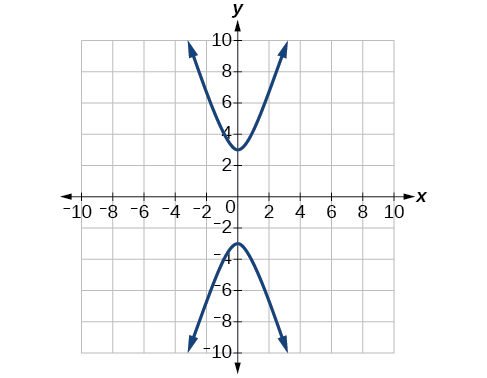$\frac{{\left(x-2\right)}^{2}}{16}-\frac{{\left(y+3\right)}^{2}}{25}=1$

$-4{x}^{2}-16x+{y}^{2}-2y-19=0$

$y\left(x\right)=1+2\sqrt{{x}^{2}+4x+5},y\left(x\right)=1-2\sqrt{{x}^{2}+4x+5}$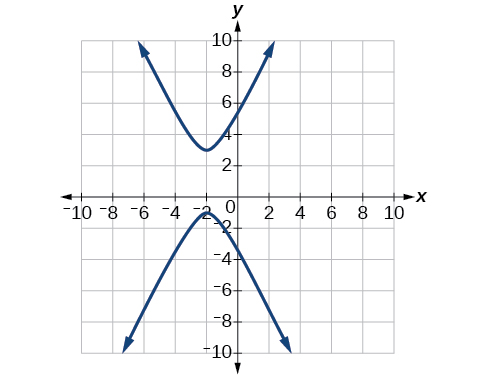$4{x}^{2}-24x-{y}^{2}-4y+16=0$

## Real-world applications

For the following exercises, a hedge is to be constructed in the shape of a hyperbola near a fountain at the center of the yard. Find the equation of the hyperbola and sketch the graph.

The hedge will follow the asymptotes and its closest distance to the center fountain is 5 yards.

$\frac{{x}^{2}}{25}-\frac{{y}^{2}}{25}=1$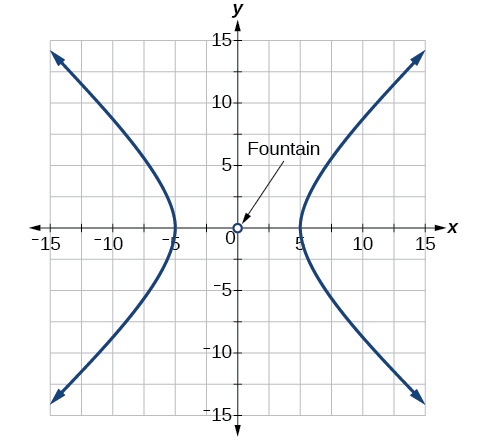The hedge will follow the asymptotes and its closest distance to the center fountain is 6 yards.

The hedge will follow the asymptotes $\text{\hspace{0.17em}}y=\frac{1}{2}x\text{\hspace{0.17em}}$ and $\text{\hspace{0.17em}}y=-\frac{1}{2}x,$ and its closest distance to the center fountain is 10 yards.

$\frac{{x}^{2}}{100}-\frac{{y}^{2}}{25}=1$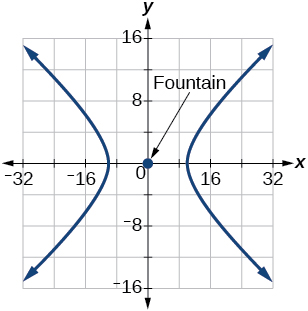The hedge will follow the asymptotes $\text{\hspace{0.17em}}y=\frac{2}{3}x\text{\hspace{0.17em}}$ and $\text{\hspace{0.17em}}y=-\frac{2}{3}x,$ and its closest distance to the center fountain is 12 yards.

The hedge will follow the asymptotes and its closest distance to the center fountain is 20 yards.

$\frac{{x}^{2}}{400}-\frac{{y}^{2}}{225}=1$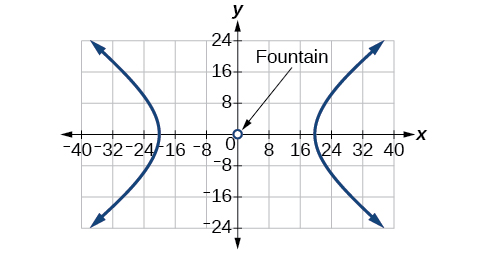For the following exercises, assume an object enters our solar system and we want to graph its path on a coordinate system with the sun at the origin and the x-axis as the axis of symmetry for the object's path. Give the equation of the flight path of each object using the given information.

The object enters along a path approximated by the line $\text{\hspace{0.17em}}y=x-2\text{\hspace{0.17em}}$ and passes within 1 au (astronomical unit) of the sun at its closest approach, so that the sun is one focus of the hyperbola. It then departs the solar system along a path approximated by the line $\text{\hspace{0.17em}}y=-x+2.\text{\hspace{0.17em}}$

The object enters along a path approximated by the line $\text{\hspace{0.17em}}y=2x-2\text{\hspace{0.17em}}$ and passes within 0.5 au of the sun at its closest approach, so the sun is one focus of the hyperbola. It then departs the solar system along a path approximated by the line $\text{\hspace{0.17em}}y=-2x+2.\text{\hspace{0.17em}}$

$\frac{{\left(x-1\right)}^{2}}{0.25}-\frac{{y}^{2}}{0.75}=1$

The object enters along a path approximated by the line $\text{\hspace{0.17em}}y=0.5x+2\text{\hspace{0.17em}}$ and passes within 1 au of the sun at its closest approach, so the sun is one focus of the hyperbola. It then departs the solar system along a path approximated by the line $\text{\hspace{0.17em}}y=-0.5x-2.\text{\hspace{0.17em}}$

The object enters along a path approximated by the line $\text{\hspace{0.17em}}y=\frac{1}{3}x-1\text{\hspace{0.17em}}$ and passes within 1 au of the sun at its closest approach, so the sun is one focus of the hyperbola. It then departs the solar system along a path approximated by the line

$\frac{{\left(x-3\right)}^{2}}{4}-\frac{{y}^{2}}{5}=1$

The object It enters along a path approximated by the line $\text{\hspace{0.17em}}y=3x-9\text{\hspace{0.17em}}$ and passes within 1 au of the sun at its closest approach, so the sun is one focus of the hyperbola. It then departs the solar system along a path approximated by the line $\text{\hspace{0.17em}}y=-3x+9.\text{\hspace{0.17em}}$

what are odd numbers
numbers that leave a remainder when divided by 2
Thorben
1,3,5,7,... 99,...867
Thorben
the third and the seventh terms of a G.P are 81 and 16, find the first and fifth terms.
if a=3, b =4 and c=5 find the six trigonometric value sin
pls how do I factorize x⁴+x³-7x²-x+6=0
in a function the input value is called
how do I test for values on the number line
if a=4 b=4 then a+b=
a+b+2ab
Kin
commulative principle
a+b= 4+4=8
Mimi
If a=4 and b=4 then we add the value of a and b i.e a+b=4+4=8.
Tariq
what are examples of natural number
an equation for the line that goes through the point (-1,12) and has a slope of 2,3
3y=-9x+25
Ishaq
show that the set of natural numberdoes not from agroup with addition or multiplication butit forms aseni group with respect toaaddition as well as multiplication
x^20+x^15+x^10+x^5/x^2+1
evaluate each algebraic expression. 2x+×_2 if ×=5
if the ratio of the root of ax+bx+c =0, show that (m+1)^2 ac =b^2m
By the definition, is such that 0!=1.why?
(1+cosA+IsinA)(1+cosB+isinB)/(cos@+isin@)(cos$+isin$)
hatdog
Mark
jaks
Ryan

#### Get Jobilize Job Search Mobile App in your pocket Now!ByByBy Madison ChristianBy Vanessa SoledadBy Brooke DelaneyBy Stephen VoronBy OpenStaxBy Angela EckmanBy Jordon HumphreysBy OpenStaxBy OpenStaxBy OpenStax